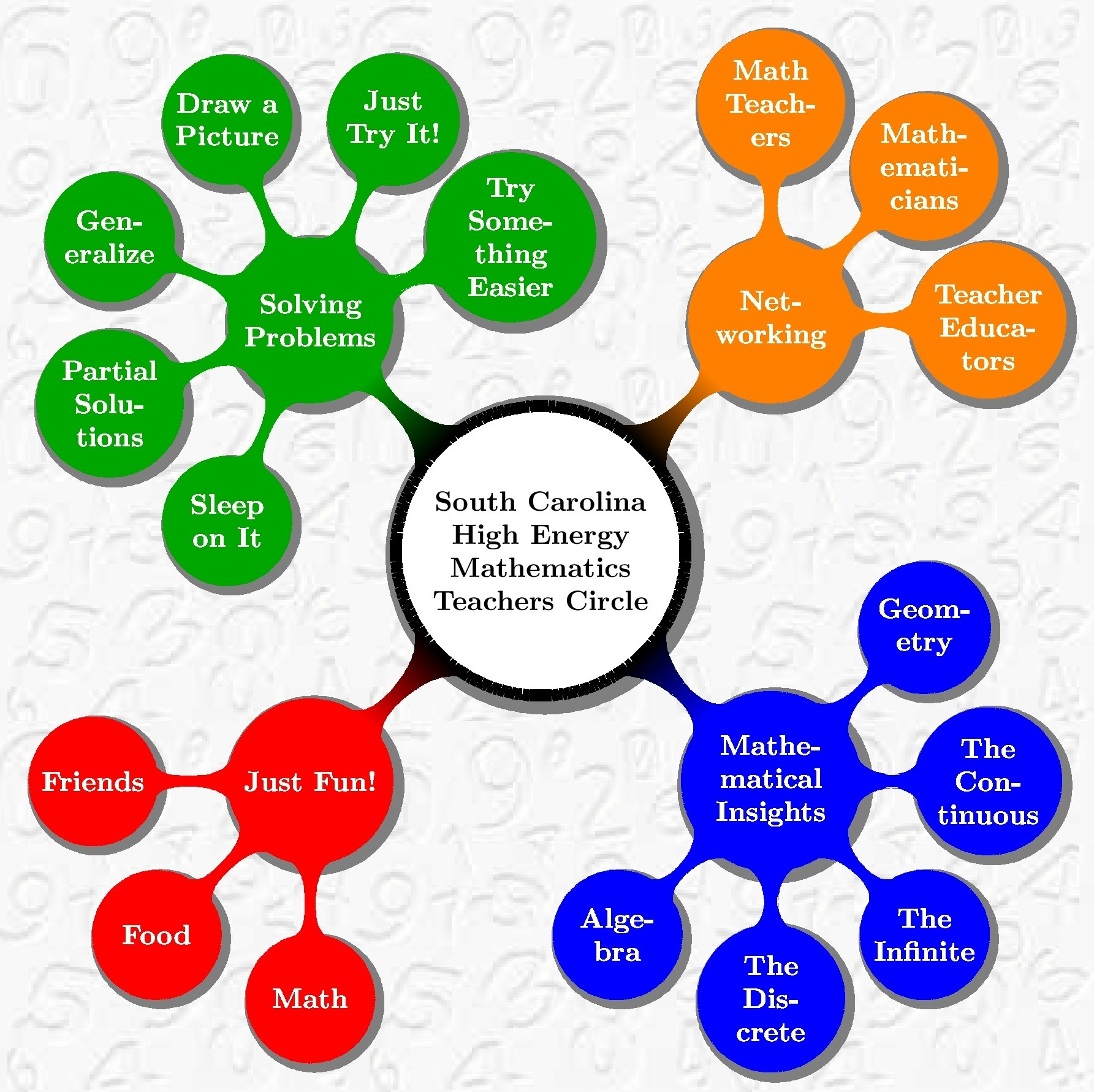South Carolina High Energy Mathematics Teachers' Circle Four Points, Six Line Segments of Two Different LengthsPut four distinct points anywhere on the plane. Connect each pair of these points with line segments. This yields six line segments (which might cross each other and some might even overlap, depending on the locations of the four points). The line segments might have very different lengths. If the four points are located at the corners of a square, then these segments will be the four sides of the square and the two diagonals, which are longer than the sides. Here are two problems to start you thinking: Are there other four point configurations on the plane, other than squares, which give rise to segments of only two different lengths? How many essentially different four point configurations on the plane can exhibit segments of just two different lengths? What does essentially different mean?